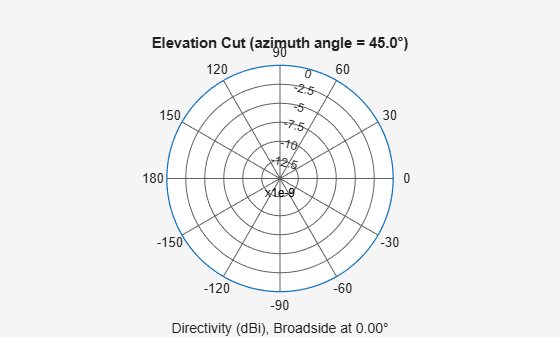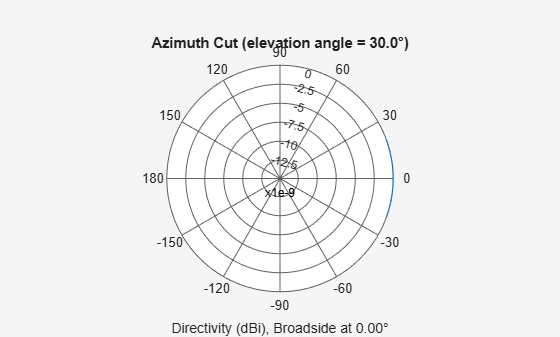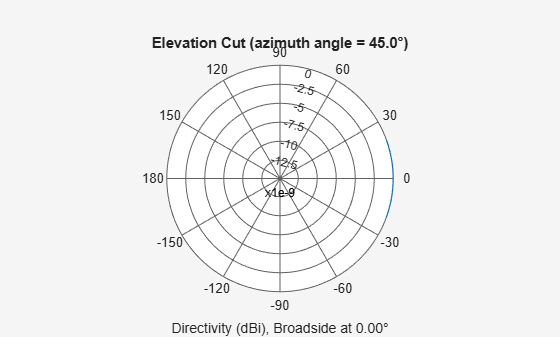# phased.IsotropicHydrophone

Isotropic hydrophone element

## Description

The `phased.IsotropicHydrophone` System object™ models an isotropic hydrophone for sonar applications. An isotropic hydrophone has the same response in all signal directions. The response is the output voltage of the hydrophone per unit sound pressure. The response of a hydrophone is also called its sensitivity. You can specify the response using the `VoltageSensitivity` property.

To compute the response of the isotropic hydrophone element for specified directions:

1. Create the `phased.IsotropicHydrophone` object and set its properties.

2. Call the object with arguments, as if it were a function.

## Creation

### Syntax

``hydrophone = phased.IsotropicHydrophone``
``hydrophone = phased.IsotropicHydrophone(Name=Value)``

### Description

example

````hydrophone = phased.IsotropicHydrophone` creates an isotropic hydrophone System object, `hydrophone`.```

example

````hydrophone = phased.IsotropicHydrophone(Name=Value)` creates an Isotropic hydrophone object, `hydrophone`, with each specified property set to the specified value. You can specify additional name-value pair arguments in any order as (`Name1=Value1`,...,`NameN=ValueN`).Example: ```hydrophone = phased.IsotropicHydrophone(FrequencyRange=[0 1000],BackBaffled=true)``` creates an isotropic hydrophone element with its frequency range specified between 0 and 1000 Hz.```

## Properties

expand all

Unless otherwise indicated, properties are nontunable, which means you cannot change their values after calling the object. Objects lock when you call them, and the `release` function unlocks them.

If a property is tunable, you can change its value at any time.

Operating frequency range of hydrophone, specified as a real-valued 1-by-2 row vector of the form `[LowerBound HigherBound]`. This property defines the frequency range over which the hydrophone has a response. The hydrophone element has zero response outside this frequency range. Units are in Hz.

Example: `[0 1000]`

Data Types: `double`

Voltage sensitivity of hydrophone, specified as a scalar or real-valued 1-by-K row vector. When you specify the voltage sensitivity as a scalar, that value applies to the entire frequency range specified by `FrequencyRange`. When you specify the voltage sensitivity as a vector, the frequency range is divided into K-1 equal intervals. The sensitivity values are assigned to the interval end points. The `step` method interpolates the voltage sensitivity for any frequency inside the frequency range. Units are in dB//1V/μPa. See Hydrophone Sensitivity for more details.

Example: `10`

Data Types: `double`

Baffle the back direction of hydrophone element, specified as `false` or `true`. When `true`, the hydrophone responses to all azimuth angles beyond ±90 degrees from broadside (zero degrees azimuth and elevation) are zero.

When the value of this property is `false`, the back direction of the microphone element is not baffled.

## Usage

### Syntax

``resp = hydrophone(freq,ang)``

### Description

````resp = hydrophone(freq,ang)` returns the voltage sensitivity for the hydrophone at the specified operating frequencies and in the specified directions of arriving signals.```

### Input Arguments

expand all

Voltage sensitivity frequencies of hydrophone, specified as a positive real scalar or a real-valued 1-by-L vector of positive values. Units are in Hz.

Data Types: `double`

Direction of arriving signals, specified as a real-valued 1-by-M row vector or 2-by-M matrix. When `ang` is a 2-by-M matrix, each column of the matrix specifies the direction in the form `[azimuth;elevation]`. The azimuth angle must lie between –180° and 180°, inclusive. The elevation angle must lie between –90° and 90°, inclusive.

When `ang` is a 1-by-M row vector, each element specifies the azimuth angle of the arriving signal. In this case, the corresponding elevation angle is assumed to be zero.

Data Types: `double`

### Output Arguments

expand all

Voltage sensitivity of hydrophone, returned as a real-valued M-by-L matrix. M represents the number of angles specified in `ang`, and L represents the number of frequencies specified in `freq`. Units are in V/Pa.

## Object Functions

To use an object function, specify the System object as the first input argument. For example, to release system resources of a System object named `obj`, use this syntax:

`release(obj)`

expand all

 `beamwidth` Compute and display beamwidth of sensor element pattern `directivity` Directivity of antenna or transducer element `isPolarizationCapable` Antenna element polarization capability `pattern` Plot antenna or transducer element directivity and patterns `patternAzimuth` Plot antenna or transducer element directivity and pattern versus azimuth `patternElevation` Plot antenna or transducer element directivity and pattern versus elevation
 `step` Run System object algorithm `release` Release resources and allow changes to System object property values and input characteristics `reset` Reset internal states of System object

## Examples

collapse all

Compute the response of an isotropic hydrophone operating at 2 kHz. The hydrophone has default property values.

Obtain the voltage sensitivity at five different elevation angles: ${-30}^{\circ }$, ${-15}^{\circ }$, ${0}^{\circ }$, ${15}^{\circ }$ and ${30}^{\circ }$. All azimuth angles are at ${0}^{\circ }$. The sensitivities are computed at the signal frequency of 2 kHz.

```hydrophone = phased.IsotropicHydrophone; fc = 2e3; resp = hydrophone(fc,[0 0 0 0 0;-30 -15 0 15 30]);```

Examine the response and patterns of an isotropic hydrophone operating between 1 kHz and 10 kHz.

Set up the hydrophone parameters. Obtain the voltage sensitivity at five different elevation angles: ${-30}^{\circ }$, ${-15}^{\circ }$, ${0}^{\circ }$, ${15}^{\circ }$ and ${30}^{\circ }$. All azimuth angles are at ${0}^{\circ }$. The sensitivities are computed at the signal frequency of 2 kHz.

```hydrophone = phased.IsotropicHydrophone('FrequencyRange', ... [1 10]*1e3); fc = 2e3; resp = hydrophone(fc,[0 0 0 0 0;-30 -15 0 15 30]);```

Draw a 3-D plot of the voltage sensitivity.

```pattern(hydrophone,fc,[-180:180],[-90:90], ... 'CoordinateSystem','polar','Type','powerdb')```Examine the response and patterns of an isotropic hydrophone at three different frequencies. The hydrophone operates between 1 kHz and 10 kHz. Specify the voltage sensitivity as a vector.

Set up the hydrophone parameters and obtain the voltage sensitivity at 45° azimuth and 30° elevation. Compute the sensitivities at the signal frequencies of 2, 5, and 7 kHz.

```hydrophone = phased.IsotropicHydrophone('FrequencyRange',[1 10]*1e3, ... 'VoltageSensitivity',[-100 -90 -100]); fc = [2e3 5e3 7e3]; resp = hydrophone(fc,[45;30])```
```resp = 1×3 14.8051 29.2202 24.4152 ```

Draw a 2-D plot of the voltage sensitivity as a function of azimuth.

```pattern(hydrophone,fc,[-180:180],0,'CoordinateSystem','rectangular',... 'Type','power')```Compute the directivity of an isotropic hydrophone in different directions. Assume the signal frequency is 3 kHz. First, set up the hydrophone parameters.

```fc = 3e3; hydrophone = phased.IsotropicHydrophone('FrequencyRange',[1,10]*1e3, ... 'VoltageSensitivity',[-100,-90,-100]); patternElevation(hydrophone,fc,45)```First, select the angles of interest to be constant elevation angle at zero degrees. The five azimuth angles are centered around boresight (zero degrees azimuth and zero degrees elevation).

`ang = [-20,-10,0,10,20; 0,0,0,0,0];`

Compute the directivity along the constant elevation cut.

`d = directivity(hydrophone,fc,ang)`
```d = 5×1 0 0 0 0 0 ```

The directivity of an isotropic hydrophone is zero in every direction.

Examine the response and patterns of an isotropic hydrophone at three different frequencies. The hydrophone operates between 1 kHz and 10 kHz. Specify the voltage sensitivity as a vector.

Set up the hydrophone parameters and obtain the voltage sensitivity at 45° azimuth and 30° elevation. Compute the sensitivities at the signal frequencies of 2, 5, and 7 kHz.

```hydrophone = phased.IsotropicHydrophone('FrequencyRange',[1 10]*1e3, ... 'VoltageSensitivity',[-100 -90 -100]); fc = [2e3 5e3 7e3]; resp = hydrophone(fc,[45;30])```
```resp = 1×3 14.8051 29.2202 24.4152 ```

Draw a 2-D plot of the voltage sensitivity as a function of azimuth.

```pattern(hydrophone,fc,[-180:180],0,'CoordinateSystem','rectangular',... 'Type','power')```Examine the azimuth pattern of an isotropic hydrophone at 30° elevation. The frequency range is between 1 kHz and 10 kHz. Specify the voltage sensitivity as a vector.

First, set up the hydrophone parameters.

```fc = 3e3; hydrophone = phased.IsotropicHydrophone('FrequencyRange',[1,10]*1e3, ... 'VoltageSensitivity',[-100,-90,-100]); patternAzimuth(hydrophone,fc,30)```Plot a smaller range of azimuth angles using the `Azimuth` parameter.

`patternAzimuth(hydrophone,fc,30,'Azimuth',[-20:20])`Plot an elevation cut of directivity of an isotropic hydrophone at 45° azimuth. Assume the signal frequency is 3 kHz. First, set up the hydrophone parameters.

```fc = 3e3; hydrophone = phased.IsotropicHydrophone('FrequencyRange',[1,10]*1e3, ... 'VoltageSensitivity',[-100,-90,-100]); patternElevation(hydrophone,fc,45)```Plot a smaller range of elevation angles using the `Elevation` parameter.

`patternElevation(hydrophone,fc,45,'Elevation',-20:20)`expand all

## Algorithms

The total sensitivity of a hydrophone is a combination of its frequency sensitivity and spatial sensitivity. `phased.IsotropicHydrophone` calculates both sensitivities using nearest neighbor interpolation, and then multiplies the sensitivities to form the total sensitivity.

 Urick, R.J. Principles of Underwater Sound. 3rd Edition. New York: Peninsula Publishing, 1996.

 Sherman, C.S., and J. Butler. Transducers and Arrays for Underwater Sound. New York: Springer, 2007.

 Allen, J.B., and D. Berkely. “Image method for efficiently simulating small-room acoustics”, Journal of the Acoustical Society of America. Vol. 65, No. 4. April 1979, pp. 943–950.

 Van Trees, H. Optimum Array Processing. New York: Wiley-Interscience, 2002, pp. 274–304.